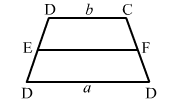# In the given figure ABCD is a trapezium in which AB || DC such that AB = a cm and DC = b cm.

Question:

In the given figure ABCD is a trapezium in which AB || DC such that AB = a cm and DC = b cm. If E and F are the midpoints of AD and BC respectively. Then, ar(ABFE) : ar(EFCD) = ?

(a) a : b
(b) (a + 3b) : (3a + b)
(c) (3a + b) : (a + 3b)
(d) (2a + b) : (3a + b)Solution:

(c) (3a +b) : (a +3b)

Clearly, EF $=\frac{1}{2}(a+b)$          [Mid point theorem]

Let d be the distance between AB and EF.
Then d is the distance between DC and EF.

Now, we have :

$\operatorname{ar}(\operatorname{trap} A B E F)=\frac{1}{2}\left(a+\frac{a+b}{2}\right) d=\frac{(3 a+b) d}{4}$

$\operatorname{ar}(\operatorname{trap} E F C D)=\frac{1}{2}\left(b+\frac{a+b}{2}\right) d=\frac{(a+3 b) d}{4}$

$\therefore$ Required ratio $=\frac{(3 a+b) d}{4}: \frac{(a+3 b) d}{4}=(3 a+b):(a+3 b)$